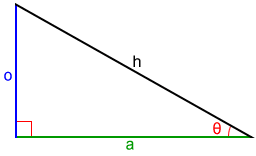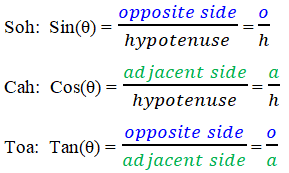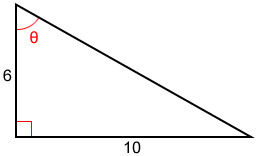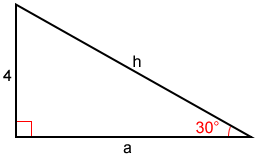# SohCahToa

SohCahToa is an acronym that serves as a mnemonic for recalling the three basic trigonometric functions: sine, cosine, and tangent.

In a right triangle, the hypotenuse, h, is the side opposite the right angle. Relative to the acute angle θ, shown below, the legs are called the opposite side, o, and the adjacent side a.By SohCahToa, the S, C, and T stand for the trigonometric functions, sine, cosine, and tangent. The three parts can be broken down as follows:The values of the trigonometric functions can be found when two sides of a right triangle are given. The third side can be found using the Pythagorean Theorem.

For a right triangle with a hypotenuse of length h and legs of length a and o:

a2 + o2 = h2

Example:

Find sin(θ), cos(θ), and tan(θ) for the right triangle below.First, find the hypotenuse, h, using the Pythagorean Theorem, where a = 6 and o = 10.Now,Example:

Find the hypotenuse and adjacent side for the right triangle below.We'll find h first.h = 8

We'll use tangent to find a next.You could also use the cosine function to find a since you have the value for c. You could even use the Pythagorean Theorem to find a since you know the other two sides of the triangle.